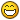## Recommended Posts

Hello,

I was checking this code (From this babylonJS tutorial: https://doc.babylonjs.com/tutorials/Ribbon_Tutorial)

``````var pi2 = Math.PI * 2;
var step = pi2 / 60;       // we want 60 points
for (var i = 0; i < pi2; i += step ) {
var x = radius * Math.sin(i);
var z = radius * Math.cos(i);
var y = 0;
path.push( new BABYLON.Vector3(x, y, z) );
}
path.push(path);       // to close the circle``````

it helped me to get points that belongs to the circumference of a circle with a specific radius, my question here is more mathematical, but I would appreciate if someone can help.

The above code assumes that the center of the circle is (0,0,0), how can I change the code in order to get the circle points assuming the center of the circle is (x1,0,z1) ?

##### Share on other sites
``````var ox = 0;
var oy = 0;
var oz = 0;

var pi2 = Math.PI * 2;
var step = pi2 / 60;       // we want 60 points
for (var i = 0; i < pi2; i += step ) {
var x = radius * Math.sin(i) + ox;
var z = radius * Math.cos(i) + oz;
var y = oy;
path.push( new BABYLON.Vector3(x, y, z) );
}
path.push(path);``````

##### Share on other sites
1 hour ago, Terminator said:

Hello,

I was checking this code (From this babylonJS tutorial: https://doc.babylonjs.com/tutorials/Ribbon_Tutorial)

```
```var pi2 = Math.PI * 2;
var step = pi2 / 60;       // we want 60 points
for (var i = 0; i < pi2; i += step ) {
var x = radius * Math.sin(i);
var z = radius * Math.cos(i);
var y = 0;
path.push( new BABYLON.Vector3(x, y, z) );
}
path.push(path);       // to close the circle``````

it helped me to get points that belongs to the circumference of a circle with a specific radius, my question here is more mathematical, but I would appreciate if someone can help.

The above code assumes that the center of the circle is (0,0,0), how can I change the code in order to get the circle points assuming the center of the circle is (x1,0,z1) ?

``````var ox = 0;
var oy = 0;
var oz = 0;

var tau = Math.PI * 2;
var step = tau / 60;       // we want 60 points
for (var i = 0; i < tau; i += step ) {
var x = radius * Math.sin(i) + ox;
var z = radius * Math.cos(i) + oz;
var y = oy;
path.push( new BABYLON.Vector3(x, y, z) );
}
path.push(path);``````

##### Share on other sites

hahaha @symof !! cool video##### Share on other sites

i just type 44 same thing, in most cases. saving bytes or not copy+paste #defines from somewhere( over and over again) makes me happy

## Join the conversation

You can post now and register later. If you have an account, sign in now to post with your account.
Note: Your post will require moderator approval before it will be visible.×   Pasted as rich text.   Paste as plain text instead

Only 75 emoji are allowed.